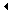Instrument display warnings

# Instrument display warnings

Discussion

Original Poster1,290 posts

205 months

I have recently started get 'warning efi fault code 04' flashing up on the instrument pod.
I have had a look through my Sagaris workshop manual, but cannot find any references to the fault codes.
Is there list available anywhere referencing fault codes.....

1,882 posts

155 months

Here you go:

Warning Codes:

Oil_P_Under_Min =0x01 =1
Oil_P_Over_Max =0x02 =2
Oil_P_Lamp_On =0x03 =3
EFI_MIL_Lamp_On =0x04 =4
Water_T_Over_Max =0x05 =5
Oil_T_Over_Max =0x06 =6
Battery_Over_Max =0x07 =7
Alternator_Lamp_On =0x08 =8
Inertia_Lamp_On =0x09 =9
Battery_Under_Min =0x0a =10
Fuel_L_Under_Min =0x0b =11
Air_T_Under_Min =0x0c =12
Brake_Fluid_Lamp_On =0x0d =13
RH_Door_Lamp_On =0x0e =14
LH_Door_Lamp_On =0x0f =15
Light left on =0x10 =16
No seat belt =0x11 =17
Gear_T_Over_Max =0x12 =18
Diff_T_Over_Max =0x13 =19
Low_Fuel_Pressure =0x14 =20
High_Fuel_Pressure =0x15 =21

Fault Codes:

Water sensor =0x80 =128
Oil temp sensor =0x81 =129
Oil pressure sensor =0x82 =130
Fuel level sensor =0x83 =131
Air temp sensor =0x84 =132
Speedo zero =0x85 =133
Tacho zero =0x86 =134
Heater controls =0x87 =135
Heater flap zero =0x88 =136
Aircon Fault =0x89 =137
Gear_T_Sensor_Fault =0x8a =138
Diff_T_Sensor_Fault =0x8b =139
Fuel_P_Sensor_Fault =0x8c =140
Brake Fluid Sw.fault =0x8d =141

Power supply control =0x90 =144
Display FRAM failed =0x91 =145
Display FRAM corrupt =0x92 =146
Stepper FRAM failed =0x93 =147
Stepper FRAM corrupt =0x94 =148
Time clock failed =0x95 =149
Time clock corrupt =0x96 =150
Inter uP comms failed =0x97 =151
System initiation =0x98 =152
Software invalid =0x99 =153
Display switches fault =0x9a =154

Original Poster1,290 posts

205 months

Excellent thank you very much.

So its a 'EFI_MIL_Lamp_On' !!!!

HMMMM interesting, I thought only the V8 Cerbera hand a MIL Lamp ????

Unless its mean something completely different on my car.....

Edited by zooooom on Tuesday 10th September 20:57

1,882 posts

155 months

It means the same as the Cerb Mil light, i.e. the engine ECU is reporting an issue. Having said that, many of the 'T' cars report this 'fault' when first starting but it disappears after a couple of seconds.

966 posts

148 months

I get this one every-time I start the T350; no idea why.. and it's been 4 years of them

I also got it about 50% of the time on the Sagaris, again no idea why.. just seemed to be a quirk..

I'd love to know the reason but unless someone can tell me it's a bad thing I'll just keep ignoring it!